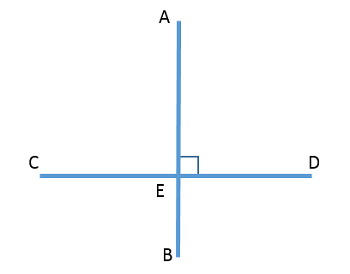# Mathematical Proof: Definition & Examples

An error occurred trying to load this video.

Try refreshing the page, or contact customer support.

Coming up next: Perpendicular Slope: Definition & Examples

### You're on a roll. Keep up the good work!

Replay
Your next lesson will play in 10 seconds
• 0:03 Definitions
• 1:09 Paragraph Proofs
• 1:56 Flow Chart Proofs
• 2:47 Two-Column Proofs
• 3:15 Lesson Summary

Want to watch this again later?

Timeline
Autoplay
Autoplay
Speed

#### Recommended Lessons and Courses for You

Lesson Transcript
Instructor: Mia Primas

Mia has taught math and science and has a Master's Degree in Secondary Teaching.

Have you ever made a statement that someone challenged you to prove to be true? You have to explain things in a logical and indisputable way in order to do that. In this lesson, you'll learn different ways that statements can be proven in mathematics.

## Definitions

Writing a mathematical proof is similar to an attorney arguing a case in a courtroom. An attorney's task is to prove a person's guilt or innocence using evidence and logical reasoning. A mathematical proof shows a statement to be true using definitions, theorems, and postulates. Just as with a court case, no assumptions can be made in a mathematical proof. Every step in the logical sequence must be proven. Mathematical proofs use deductive reasoning, where a conclusion is drawn from multiple premises. The premises in the proof are called statements.

Proofs can be direct or indirect. In a direct proof, the statements are used to prove that the conclusion is true. An indirect proof, on the other hand, is a proof by contradiction. It begins by assuming the opposite of the statement that is to be proven. During the proof, a contradiction will be reached, showing that the assumed statement is false. For the examples in this lesson, we will use direct proofs since they are used more commonly.

The format of a proof can be a simple paragraph, a flow chart, or a two-column chart. We will look at an example of each.

## Paragraph Proofs

All proofs should begin with the given information that is provided. When writing a paragraph proof, each sentence provides a statement and explanation leading to the conclusion. The conclusion is the statement that is being proven.

For example:

• Given that angle AED is a right angle, prove that angle AEC measures ninety degrees.We are given that angle AED is a right angle. According to the definition of right angles, it's therefore ninety degrees. Angle AED and angle AEC are linear and supplementary, based on the definition of linear angles. If they are supplementary, then they have a sum of 180 degrees. Therefore, since AED is ninety degrees, angle AEC must also be ninety degrees.

Next, we'll see how this proof can be made using a flow chart.

To unlock this lesson you must be a Study.com Member.

### Register to view this lesson

Are you a student or a teacher?

### Unlock Your Education

#### See for yourself why 30 million people use Study.com

##### Become a Study.com member and start learning now.
Back
What teachers are saying about Study.com

### Earning College Credit

Did you know… We have over 160 college courses that prepare you to earn credit by exam that is accepted by over 1,500 colleges and universities. You can test out of the first two years of college and save thousands off your degree. Anyone can earn credit-by-exam regardless of age or education level.